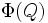# Invariant special subgroup lemma

## Statement

Suppose$P$ is a finite$p$-group, i.e., a group of prime power order. Suppose$A$ is a subgroup of$\operatorname{Aut}(P)$ such that$p$ does not divide the order of$A$. Suppose$\varphi$ is a non-identity element of$A$. Then, there exists a subgroup$Q$ of$P$ that is either special or elementary Abelian, with the following properties:

•$\varphi$ acts nontrivially on$Q/\Phi(Q)$.
•$A$ acts irreducible on$Q/\Phi(Q)$.
•$\varphi$ acts trivially on$\Phi(Q)$.

## Facts used

1. Structure lemma for p-group with coprime automorphism group having automorphism trivial on invariant subgroups

## Proof

Let$Q$ be minimal among the nontrivial$A$-invariant subgroups of$P$ on which$\varphi$ acts nontrivially. (Note that this collection of subgroups is nonempty, since$\varphi$ acts nontrivially on$P$). Fact (1) then yields that$Q$ satisfies the required conditions.